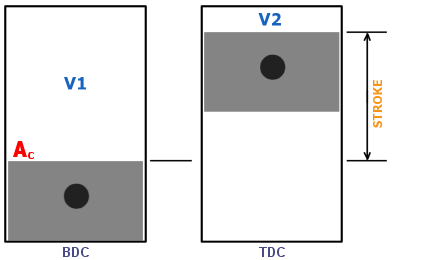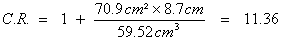Compression Ratio
 Page 1 / Page 2
A definition
 Most automotive enthusiasts are familiar with the concept of compression ratio. We know that in general a high compression ratio is good for performance and efficiency, but that it can also lead to "knocking" or "detonation". Therefore high compression ratios are usually associated with the requirement for high octane fuel and careful engine management. But there is more to it than that. Compression ratio is also dependent on cam duration, as we shall see. Let's begin by reviewing the definition of compression ratio. We will also investigate how basic engine dimensions affect the static compression ratio.The schematic on the left shows the parameters that go into calculating an engine's static compression ratio. Compression ratio if defined quite simply as the volume above the piston at bottom-dead-center (BDC), divided by the volume above the piston at top-dead-center (TDC). C.R. = V1 / V2 The volumes involved are the volume of the combustion chamber (V2) and the "swept volume" of the cylinder. The swept volume is obtained by multiplying the cross-sectional area of the cylinder bore (Ac) by the stroke. Thus the compression ratio can be written as:On my 2.5L S14 the total volume of the combustion chamber (including valve reliefs, piston pop-up and head gasket thickness) is V2 = 59.52 c.c. The stroke is 87.0 mm and the bore is 95.0 mm . Thus Ac = 70.9 cm˛ . If we plug all of this into the equation above we get:Thus my compression ratio is 11.36:1 Now if we look carefully at the equation for compression ratio above we note some interesting trends. Even if the volume of the combustion chamber (V2) is kept constant, we can increase the compression ratio simply by increasing the stroke and/or the bore size. So let's say you rebuild your engine, but all you do is bore it out slightly and install identically shaped, but bigger pistons. You don't shave the head or change the stroke, or decrease the volume of the combustion chamber. You will still increase your compression ratio. Or let's say you rebuild your engine but all you do is add a stroker crank. And you also order new pistons with the pins mounted farther up so that the piston does not intrude farther into the combustion chamber at TDC. Thus your combustion chamber volume is unchanged - all that changes is the stroke. You will still increase your compression ratio, as the equation points out. None of this is rocket science really. But it is interesting nonetheless, and might not be noticed without a careful examination of the compression ratio equation. Next page »
 Page 1 / Page 2